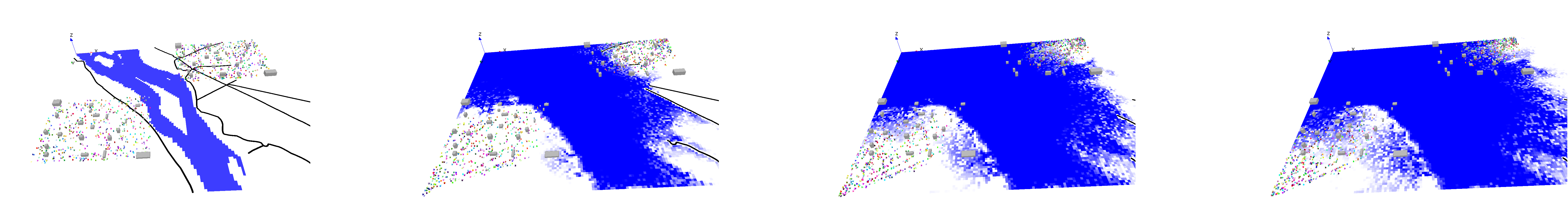Version: 1.8.1

# Using Comodel

## Introduction​

In the trend of developing a complex system of multi-disciplinary, composing and coupling models are days by days becoming the most attractive research objectives. GAMA is supporting the co-modeling and co-simulation which are supposed to be a common coupling infrastructure.

## Example of a Comodel​

A Comodel is a model, especially an agent-based model, composed of several sub-models, called micro-models. A comodel itself could be also a micro-model of another comodel. From the point of view of a micro-model, the comodel is called a macro-model.

A micro-model must be imported, instantiated, and life-controlled by a macro-model.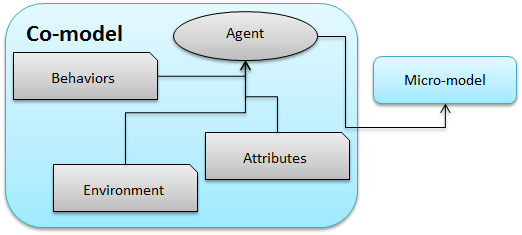## Why and when can we use Comodel?​

Co-models ca definitely be very useful when the whole model can be decomposed in several sub-models, each of them representing, in general, a dynamics of the whole model, and that interact through some entities of the model. In particular, it allows several modelers to develop the part of the model dedicated to their expertise field, to test it extensively, before integrating it inside the whole model (where integration tests should not be omitted!).

## Use of Comodel in a GAML model​

The GAML language has evolved by extending the import section. The old importation told the compiler to merge all imported elements into as one model, but the new one allows modelers to keep the elements coming from imported models separately from the caller model.

### Definition of a micro-model​

Defining a micro-model of comodel is to import an existing model with an alias name. The syntax is:

``import <path to the GAML model> as <identifier>``

The identifier is then become the new name of the micro-model.

As an example taken from the model library, we can write:

``import "Prey Predator Adapter.gaml" as Organism``

### Instantiation of a micro-model​

After the importation and giving an identifier, micro-model must be explicitly instantiated. It could be done by the `create` statement.

``create <micro-model identifier> . <experiment name> [optional parameter];``

The `<exeperiment name>` is an experiment inside micro-model. This syntax will generate some experiment agents and attach an implicit simulation.

Note: The creation of several instances is not multi-simulation, but multi-experiment. Modelers could create an experiment with multi-simulation by explicitly do the init inside the experiment scope.

As an example taken from the model library, we can write:

``global {    init {        //instantiate three instant of micro-model PreyPredator        create Organism.Simple number: 3 with: [shape::square(100), preyinit::10, predatorinit::1] ;    }}``

### Control micro-model life-cycle​

A micro-model can be controlled as any normal agent by asking the corresponding identifier, and also be destroyed by the `do die;` statement. And it can be recreated any time we need.

``ask (<micro-model identifier> . <experiment name>  at <number> ) . simulation {    ...}``

More generally, to schedule all the created simulations, we can do:

``reflex simulate_micro_models {    // ask all simulation do their job    ask (Organism.Simple collect each.simulation) {        do _step_;    }}``

## Visualization of the micro-model​

The micro-model species could display in comodel with the support of agent layer

``agents "name of layer" value: (<micro-model> . <experiment name> at <number>).<get List of agents>;``

As an example:

``display "Comodel display" {    agents "agentprey" value: (Organism.Simple accumulate each.get_prey());    agents "agentpredator" value: (Organism.Simple accumulate each.get_predator());}``

## Example of the comodel​

The following illustrations are taken from the model library provided with the GAMA platform.

### Urbanization model with a Traffic model​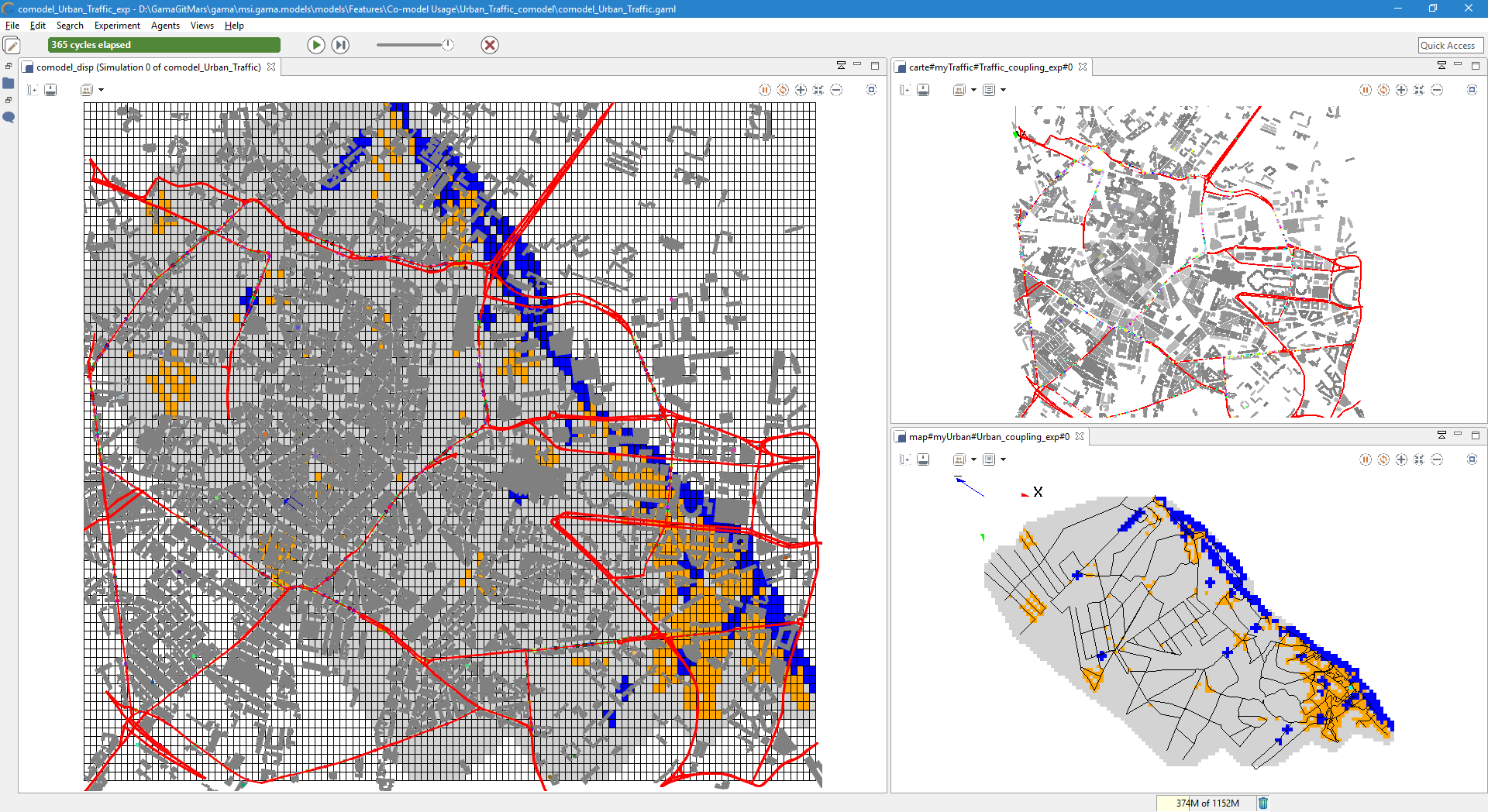### Flood model with Evacuation model​

The aim of this model is to couple the two existing models: Flood Simulation and Evacuation.

Toy Models/Evacuation/models/continuous_move.gaml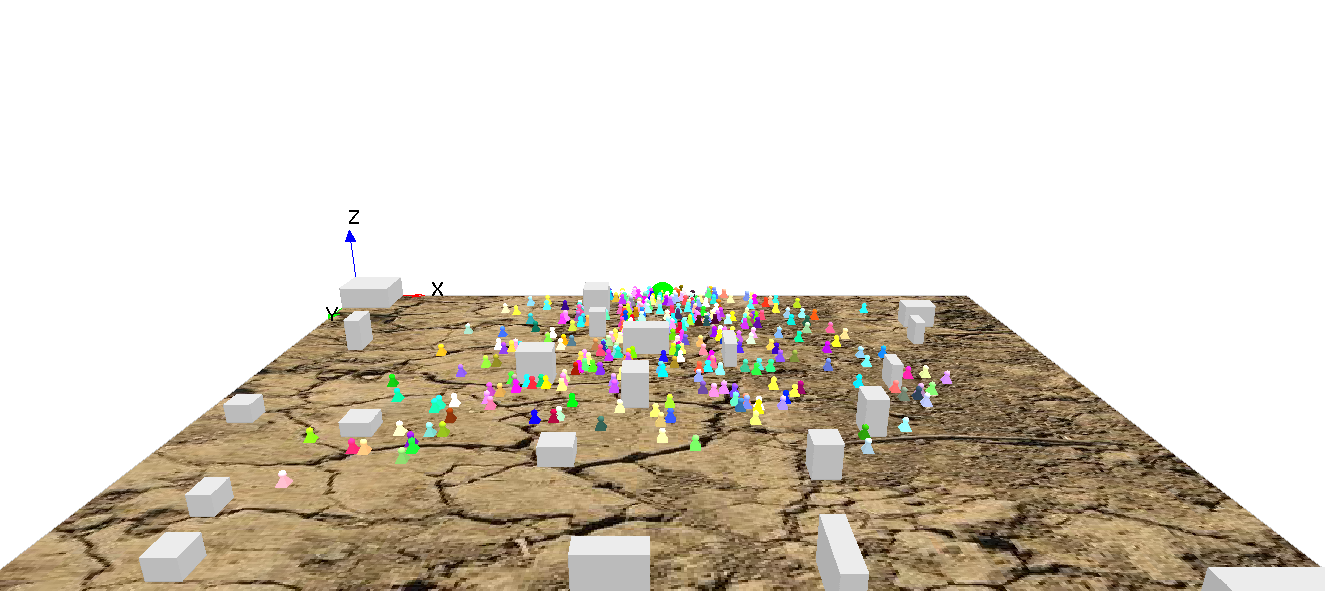Toy Models/Flood Simulation/models/Hydrological Model.gaml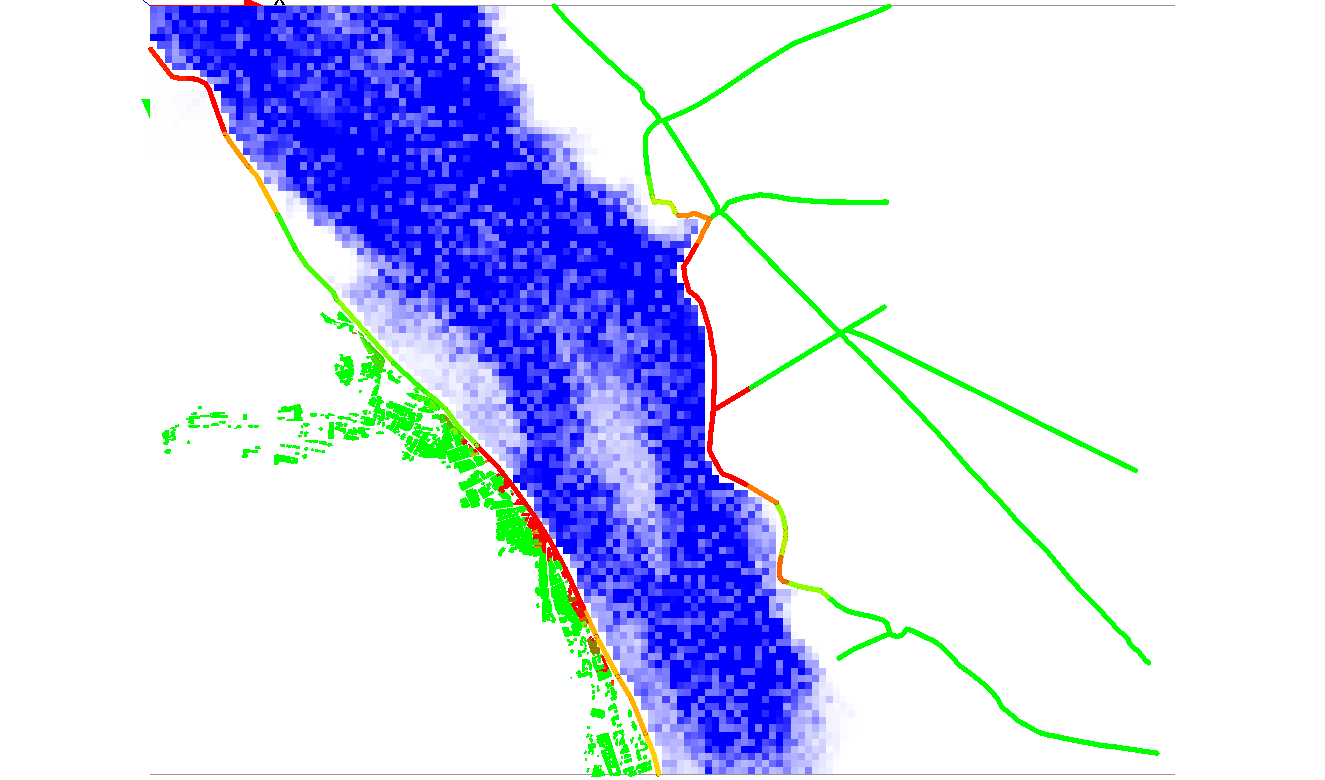The comodel explores the effect of a flood on an evacuation plan: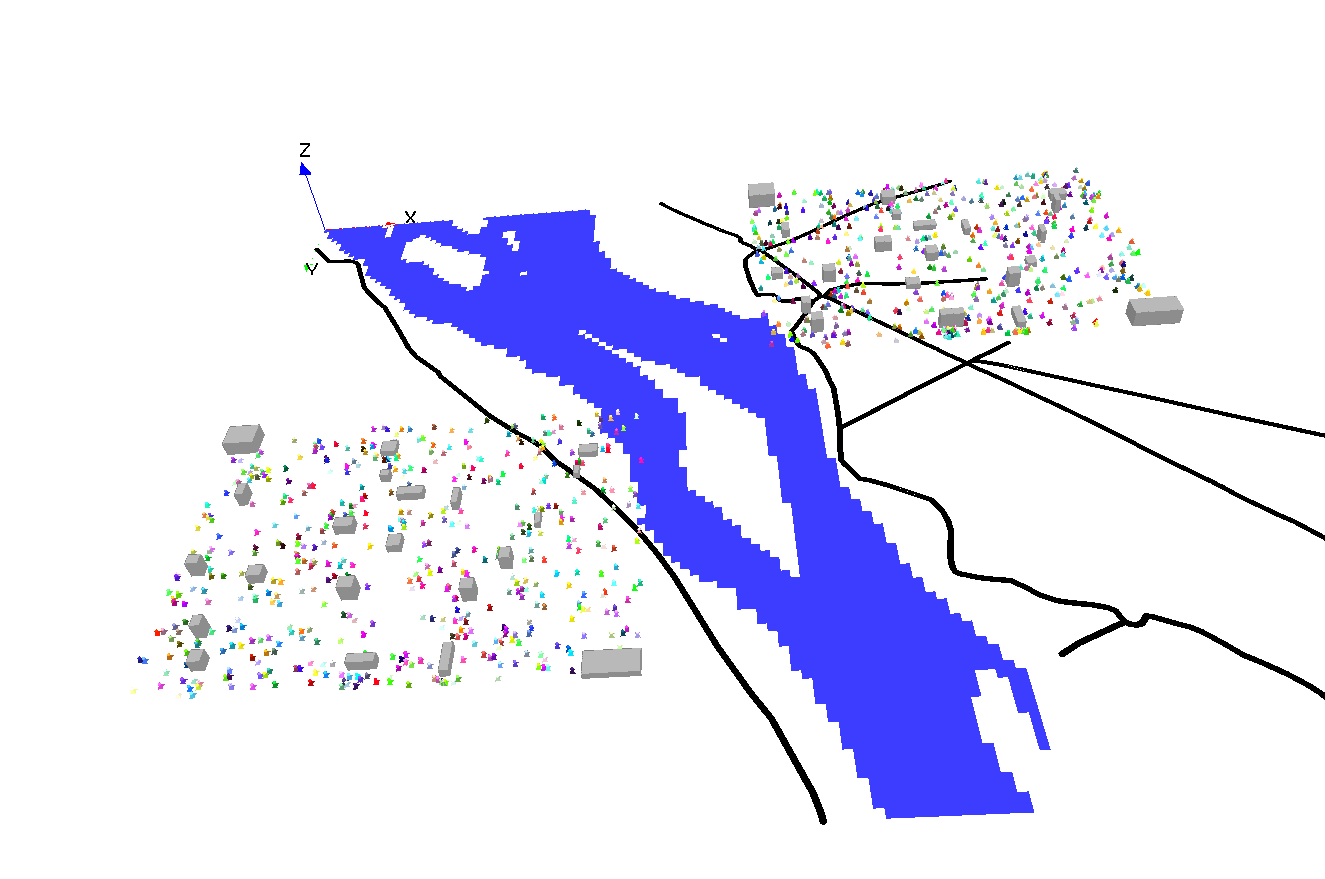Simulation results: